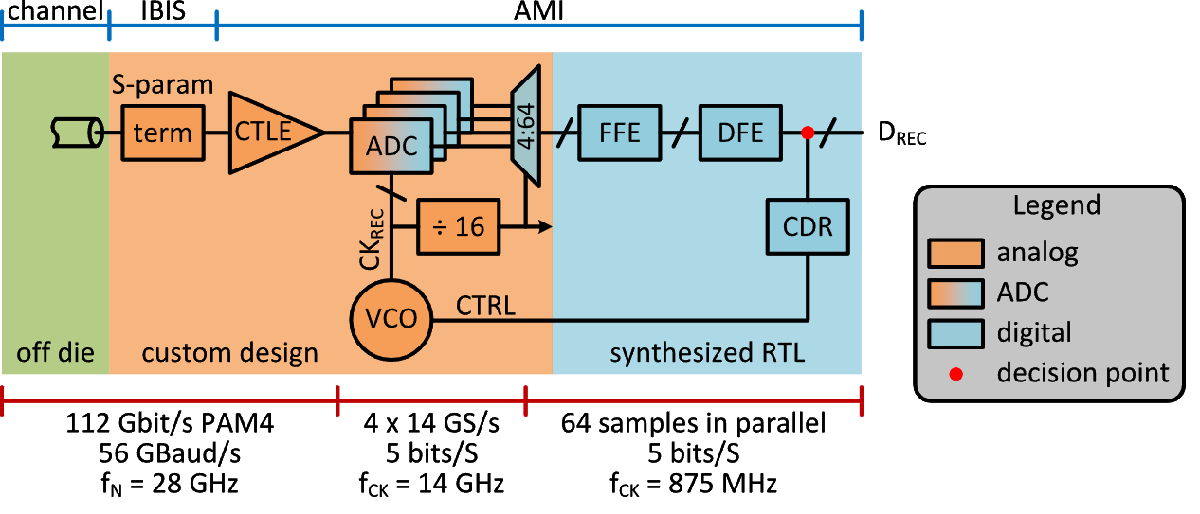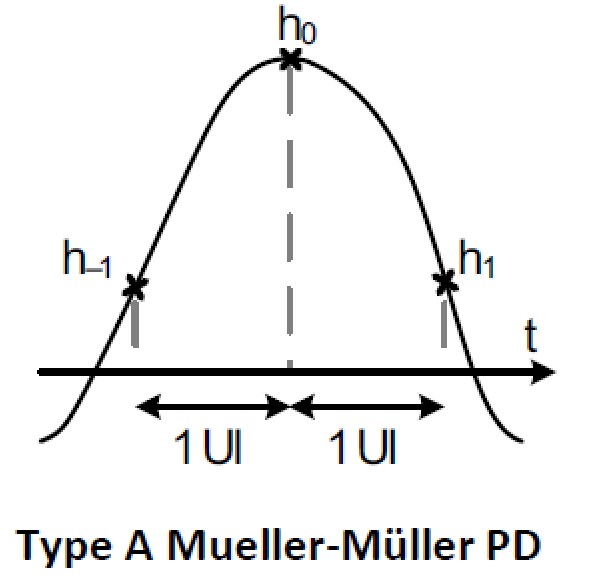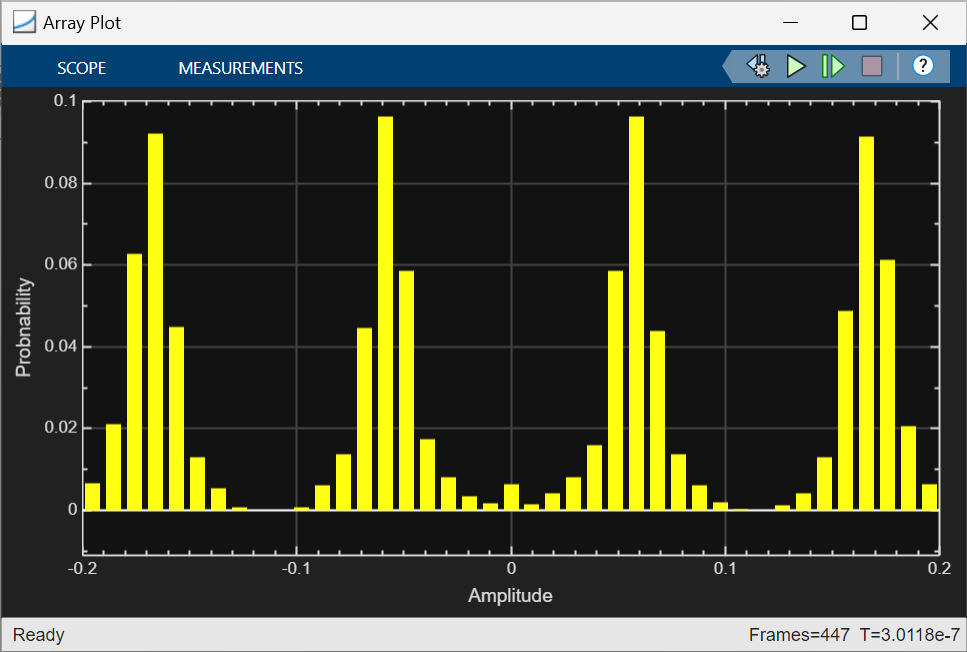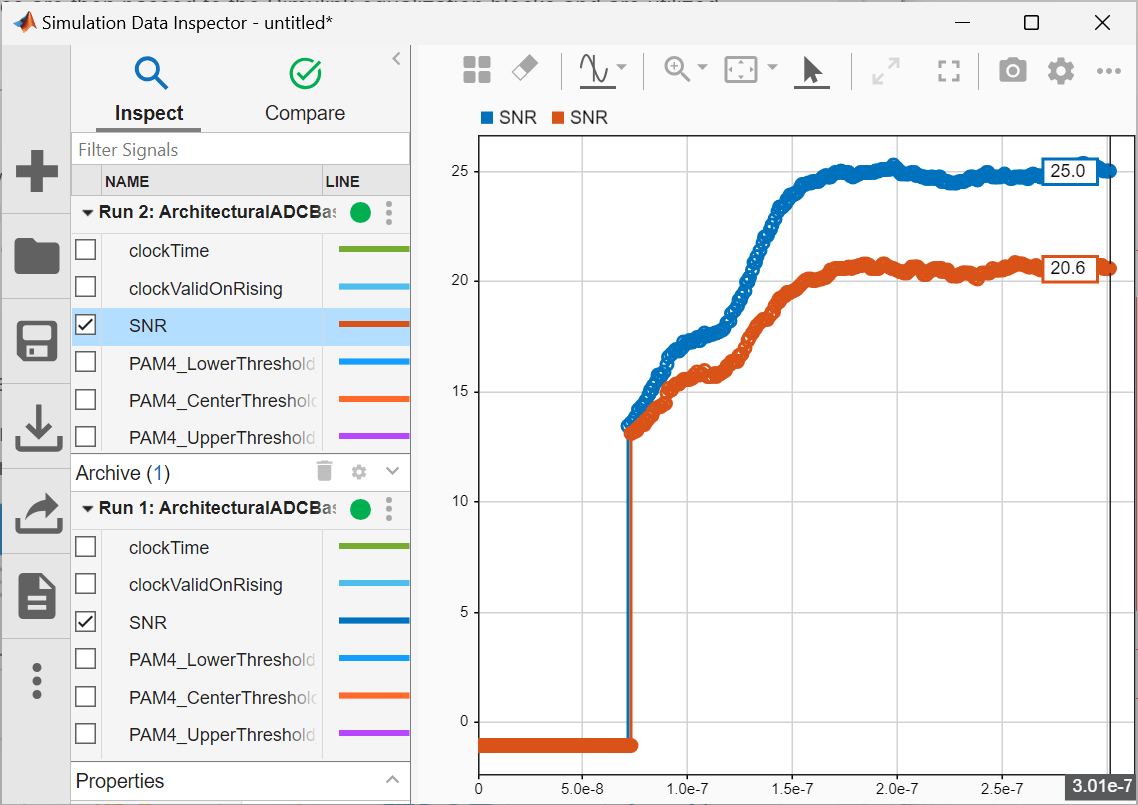# Architectural 112G PAM4 ADC-Based SerDes Model

This example demonstrates the use of a IEEE 802.3ck specification transmitter and receiver architectural model using library blocks in the SerDes Toolbox™ library and custom blocks to model a 112G PAM4 time-interleaved ADC-Based SerDes. The performance impact of the timing mismatch between the time-interleaved ADCs is explored as an example of a design tradeoff study. Those aspects of the model which are compatable with the IBIS-AMI 7.0 standard are used to create an IBIS-AMI model.

### Overview

There are many tradeoffs to be explored when designing an ADC-based SerDes. Some of these design questions are summarized below:

• What type of ADC?: Flash, binary/multibit search or SAR (successive approximation register)?

• What time-interleave factor to use? Determine system performance impact of mismatch of the timing, gain, voltage offset and bandwidth between parallel ADCs and explore mismatch calibration algorithms.

• What full scale range to use?

• Determine required resolution/quantization/number of bits.

• Explore uniform or non-uniform quantization

• What is the performance impact of quantization noise

#### Digital Equalization Exploration

• What number of FFE taps is required to achieve needed performance?

• How many DFE taps can be implimented?

• What DSP resolution is required?

• Explore impact of frame size or parallelism of digital processing on system latency. What demux width to use?

#### Clock Recovery

• What bandwidth of CDR is required? How to specify loop filter?

• Which Mueller-muller cost function is best for the application?

#### Analog Front End

• How many of CTLE stages are needed?

• How does the CTLE amplified noise impact system performance?

• Explore how to scale signal to take advantage of linear range of ADC?

This example will focus on determining the impact of the time-interleaved timing mismatch between parallel ADC but it is hoped that the model will be the basis of exploring many other design tradeoffs. The system SNR (signal-to-noise ratio) is compared for the cases with and without a 4% symbol time timing offset and shows that this impairment reduces the system performance by about 2.5 dB

### Rx Model Description

The receiver model is composed of an analog front end (AFE) with CTLE and amplifier blocks. The time-interleaved ADC is further parallized by a demux before DSP processing by the FFE and DFE. The baud-rate CDR controls the VCO which drives the ADC. The system performance is quantified by a SNR metric as well as an output waveform. This model is summarized in the following diagram where the time-interleave depth (or the number of ADC's) is four and the demux size is 64.The receiver analog front end partially equalizes the waveform and is very similar to the [link to first ADC Example ]. Here the first block injects the input refered noise, followed by the Mid-band zero CTLE, main CTLE and noise filter CTLE blocks as specified by channel operating margin (COM) of IEEE 802.3ck. The VGA scales the signal to match the full scale range of the ADC and the Saturating Amplifier block inforces a memory-nonlinearity.The ADC subsystem is composed of custom ADC, Demux, RxFFE, DFE, Phase Detector, Loop Filter and VCO system object blocks. Additionally, the IBIS-Bridge and IBIS-AMI clock_times blocks facilitate the conversion of the model to an IBIS-AMI model.A time-interleaved ADC is utilized to reduce the maximum speed and latency requirements of a full-rate ADC. The diagram below shows how four time-interleaved ADCs can take turns sampling the data signal.The sampled signals are demuxed or framed to reduce the signaling rate before processing with the DSP equalization. The diagram below illustrates how a demux width of 8 frames slows down and parallelizes the data. The model itself is parameterized to use a demux of 64 but 8 are shown below for illustration purposes only.Next a 21 tap FFE is applied to the parallized signal and then followed by the single tap DFE and data decision. The baud-rate phase detector utilizes a type A Mueller-Muller phase detector that aims to balance the ISI of the first pre and post-cursor samples as illustrated in the following graphic:The phase detector output is processed with the loop filter which in turn drives the voltage controlled oscillator (VCO) or the heart beat of the system. This VCO drives the other blocks and closes the system loop.

#### SNR Calculation

For ADC-based serdes the eye diagram is not as information rich as it is for analog SerDes, instead signal-to-noise (SNR) calculations and vertical eye slices are most useful insight into the system performance..

The DFE block calculates the SNR as follows:

`$\mathrm{SNR}=10\text{\hspace{0.17em}}{\mathrm{log}}_{10}\left(\frac{{\mu }^{2}}{{\sigma }^{2}}\right)$`

where $\mu \text{\hspace{0.17em}}$is the signal strength and $\sigma \text{\hspace{0.17em}}$is the noise strength. If $\mathit{y}\left[\mathit{i}\right]$ are the discrete equalized sampled voltages, then for NRZ the signal and noise strenths are defined as,

`$\mu =\frac{1}{\mathit{N}}\sum _{\mathit{i}=1}^{\mathit{N}}|\mathit{y}\left[\mathit{i}\right]|$`

`${\sigma }^{2}=\frac{1}{\mathit{N}}\sum _{\mathit{i}=1}^{\mathit{N}}{\left(|\mathit{y}\left[\mathit{i}\right]|-\mu \text{\hspace{0.17em}}\right)}^{2}$`

For PAM4, the signal and noise are defined as in terms of the middle and outer sampled symbol voltages.

`${\mu }_{1}=\frac{1}{{\mathit{N}}_{1}}\sum _{\mathit{i}=1}^{{\mathit{N}}_{1\text{\hspace{0.17em}}}}|\mathit{y}{\left[\mathit{i}\right]}_{\mathrm{middle}}|$`

`${\mu }_{2}=\frac{1}{{\mathit{N}}_{2}}\sum _{\mathit{i}=1}^{{\mathit{N}}_{2\text{\hspace{0.17em}}}}|\mathit{y}{\left[\mathit{i}\right]}_{\mathrm{outer}}|$`

`${\mu }^{2}=\frac{\left({\mu }_{1}^{2}+{\mu }_{2}^{2}\right)}{2}$`

`${\eta }_{1}\left[\mathit{i}\right]=\mathit{y}{\left[\mathit{i}\right]}_{\mathrm{middle}}-{\mu }_{1}$`

`${\eta }_{2}\left[\mathit{i}\right]=\mathit{y}{\left[\mathit{i}\right]}_{\mathrm{outer}}-{\mu }_{2}$`

`$\sigma {\text{\hspace{0.17em}}}^{2}=\frac{1}{{\mathit{N}}_{1}+{\mathit{N}}_{2}}\sum _{\mathit{i}}\left[{\left({\eta }_{1}\left[\mathit{i}\right]\right)}^{2}+{\left({\eta }_{2}\left[\mathit{i}\right]\right)}^{2}\right]$`

The vertical eye slice shows the clustering of the four PAM4 symbols.#### FFE and DFE Equalization Adaptation

Very similiar to the process used in Example 1 [insert link here], the FFE and DFE tap adaptation is performed at time 0 with impulse-response based analysis in the initialize subsystem. These optimized tap values are then passed to the Simulink equalization blocks and are utilized throught the simulation.

### Impact of Timing Mismatch

If the phases of the several clocks that drive the time-interleaved ADCs are not equally spaced from each other, then system performance degradation will occur. While much of this timing mismatch can be calibrated out, it is important to understand the performance impact of this impairment. The RxClock or VCO block has a parameter with the label of 'Max Timing Mismatch (UI)' as shown below. This parameter injects a phase offset between the first and second clocks. While this is a simplistic model of actual system behavior, it is sufficient to illustrate the impact.If you haven't yet, open the Simulink model 'ArchitecturalADCBasesSerDes.slx' that is in the example directory.

Run the model and observe the baseline behavior. When the simulation completes, the Simulation Data Inspector automatically loads up the logged signals

Double click on the Rx Clock / VCO block and change the timing mismatch to be 0.04 and re-run the model. The resulting SNR for the two runs can be compared as follows. This illustrates how a small 4% timing mismatch can reduce the system performance by about 2.5 dB.### Generate ADC-Based SerDes IBIS-AMI Model

The final part of this example takes the customized ADC-based SerDes Simulink model and then generates an IBIS-AMI compliant model: including model executables, IBIS and AMI files.

The current IBIS AMI standard does not have native support for ADC-based SerDes. The current standard is written for slicer-based SerDes, which contain a signal node wherein the equalized signal waveform is observed. In a slicer-based SerDes this node exists inside the DFE, right before the decision sampler. A continuous analog waveform is observable at that node, which includes the effect of all the upstream equalizers (such as CTLE) and the equalization due to DFE, as tap weighted and fed back prior decisions. Such a summing node does not exist in an ADC-based SerDes, due to the ADC in the system. In a real ADC-based SerDes system the ADC proves a vertical slice though the eye at the sampling instant. To emulate a virtual node, the IBIS-AMI Bridge block reassembles the discrete equalized samples according to the time interleave factor and the demux size. A single equalized sample is held constant for the entire IBIS-AMI waveform symbol time.

#### Export IBIS-AMI Models

Open the Export tab in the SerDes IBIS-AMI manager dialog box.

• Verify that Dual model is selected for both the Tx and the Rx AMI Model Settings. This will create model executables that support both statistical (Init) and time domain (GetWave) analysis.

• Set the Tx model Bits to ignore value to 5 since there are three taps in the Tx FFE.

• Set the Rx model Bits to ignore value to 20,000 to allow sufficient time for the Rx DFE taps to settle during time domain simulations.

• Set Models to export as Both Tx and Rx so that all the files are selected to be generated (IBIS file, AMI files and DLL files).

• Press the Export button to generate models in the Target directory.

### Explore Further

The number of ADCs and the Demux width in the model is parameterized by the MATLAB workspace variables 'timeInterleaveDepth' and 'DemuxSize'. They are set in the model PreLoadFcn callback and can be changed to other positive integers as part of further exploration. The system objects in the ADC subsystem can be modified to explore many of the design tradeoff questions identified at the first of the example.

### References

 S. Kiran, S. Cai, Y. Zhu, S. Hoyos and S. Palermo, "Digital Equalization With ADC-Based Receivers: Two Important Roles Played by Digital Signal Processingin Designing Analog-to-Digital-Converter-Based Wireline Communication Receivers," in IEEE Microwave Magazine, vol. 20, no. 5, pp. 62-79, May 2019, doi: 10.1109/MMM.2019.2898025.